Example 97.2 Box-Cox Transformations

This example shows Box-Cox transformations with a yarn failure data set. For more information about Box-Cox transformations, including using a Box-Cox transformation in a model with no independent variable, to normalize the distribution of the data, see the section Box-Cox Transformations. In this example, a simple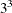design was used to study the effects of different factors on the failure of a yarn manufacturing process. The design factors are as follows:

• the length of test specimens of yarn, with levels of 250, 300, and 350 mm

• the amplitude of the loading cycle, with levels of 8, 9, and 10 mmd

• the load with levels of 40, 45, and 50 grams

The measured response was time (in cycles) until failure. However, you could just as well have measured the inverse of time until failure (in other words, the failure rate). Hence, the correct metric with which to analyze the response is not apparent. You can use PROC TRANSREG to find an optimum power transformation for the analysis. The following statements create the input SAS data set:

title 'Yarn Strength';

proc format;
value a -1 =   8 0 =   9 1 =  10;
value l -1 = 250 0 = 300 1 = 350;
value o -1 =  40 0 =  45 1 =  50;
run;

data yarn;
input Fail Amplitude Length Load @@;
format amplitude a. length l. load o.;
label fail = 'Time in Cycles until Failure';
datalines;
674 -1 -1 -1    370 -1 -1  0    292 -1 -1  1    338  0 -1 -1
266  0 -1  0    210  0 -1  1    170  1 -1 -1    118  1 -1  0
90  1 -1  1   1414 -1  0 -1   1198 -1  0  0    634 -1  0  1
1022  0  0 -1    620  0  0  0    438  0  0  1    442  1  0 -1
332  1  0  0    220  1  0  1   3636 -1  1 -1   3184 -1  1  0
2000 -1  1  1   1568  0  1 -1   1070  0  1  0    566  0  1  1
1140  1  1 -1    884  1  1  0    360  1  1  1
;

PROC TRANSREG is run to find the Box-Cox transformation. The lambda list is –2 TO 2 BY 0.05, which produces 81 lambdas, and a convenient lambda is requested. This many power parameters makes a nice graphical display with plenty of detail around the confidence interval. In the interest of space, only part of this table is displayed. The independent variables are designated with the QPOINT expansion. QPOINT, for quadratic point model, gets its name from PROC TRANSREG’s ideal point modeling capabilities, which process variables for a response surface analysis. What QPOINT does is create a set of independent variables consisting of the following: the m original variables (Length Amplitude Load), the m original variables squared (Length_2 Amplitude_2 Load_2), and the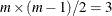pairs of products between the m variables (LengthAmplitude LengthLoad AmplitudeLoad). The following statements produce Output 97.2.1:

ods graphics on;

proc transreg details data=yarn ss2
plots=(transformation(dependent) obp);
model BoxCox(fail / convenient lambda=-2 to 2 by 0.05) =
run;

Output 97.2.1: Box-Cox Yarn Data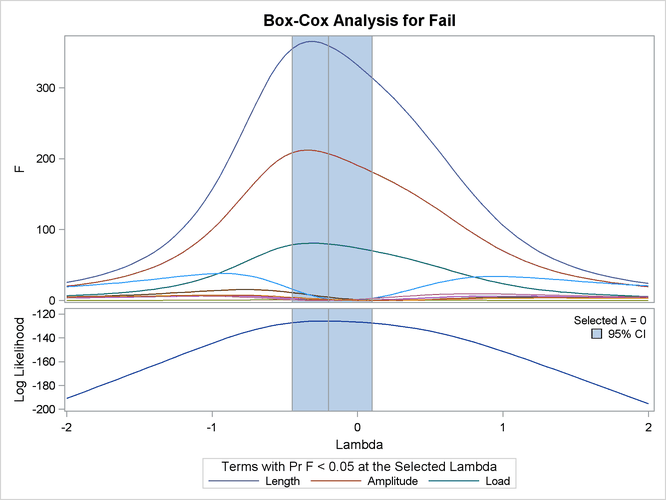Dependent Variable BoxCox(Fail)
Time in Cycles until Failure

 Number of Observations Read 27 27

Model Statement Specification Details
Type DF Variable Description Value
Dep 1 BoxCox(Fail) Lambda Used 0
Lambda -0.2
Log Likelihood -125.9
Conv. Lambda 0
Conv. Lambda LL -126.7
CI Limit -127.8
Alpha 0.05
Options Convenient Lambda Used
Label Time in Cycles until Failure
Ind 1 Qpoint.Length DF 1
Ind 1 Qpoint.Amplitude DF 1
Ind 1 Qpoint.Length_2 DF 1
Ind 1 Qpoint.Amplitude_2 DF 1
Ind 1 Qpoint.LengthAmplitude DF 1

The TRANSREG Procedure Hypothesis Tests for BoxCox(Fail)
Time in Cycles until Failure

Univariate ANOVA Table Based on the Usual Degrees of Freedom
Source DF Sum of Squares Mean Square F Value Liberal p
Model 9 22.56498 2.507220 66.73 >= <.0001
Error 17 0.63871 0.037571
Corrected Total 26 23.20369

 Root MSE R-Square 0.19383 0.9725 6.33466 0.9579 3.05987 0

Univariate Regression Table Based on the Usual Degrees of Freedom
Variable DF Coefficient Type II
Sum of
Squares
Mean Square F Value Liberal p Label
Intercept 1 6.4206207 159.008 159.008 4232.19 >= <.0001 Intercept
Qpoint.Length 1 0.8323842 12.472 12.472 331.94 >= <.0001 Length
Qpoint.Amplitude 1 -0.6309916 7.167 7.167 190.75 >= <.0001 Amplitude
Qpoint.Length_2 1 -0.0856974 0.044 0.044 1.17 >= 0.2939 Length_2
Qpoint.Amplitude_2 1 0.0242183 0.004 0.004 0.09 >= 0.7633 Amplitude_2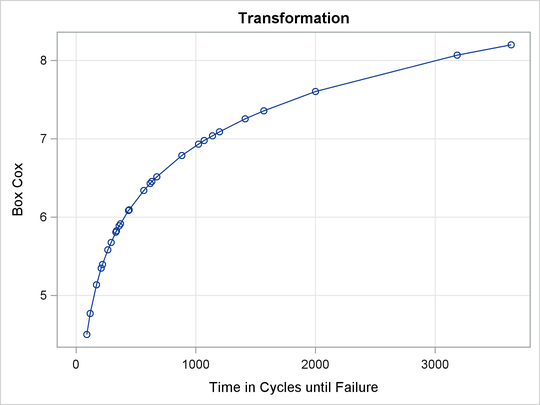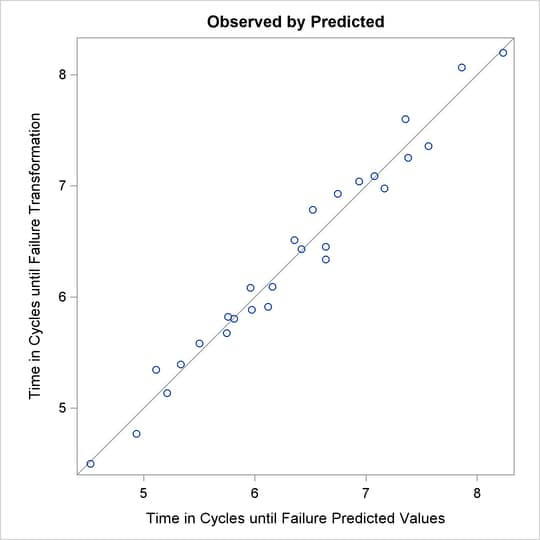The optimal power parameter is –0.20, but since 0.0 is in the confidence interval, and since the CONVENIENT t-option was specified, the procedure chooses a log transformation. The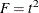plot shows in the vicinity of the optimal Box-Cox transformation, the parameters for the three original variables (Length Amplitude Load), particularly Length, are significant and the others become essentially zero.